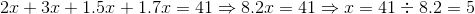# ISEE Upper Level Math : How to find the perimeter of a trapezoid

## Example Questions

### Example Question #1 : Trapezoids

The bigger base length of a trapezoid is two times bigger than the smaller base length. Legs of the trapezoid have the same length of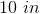. If the perimeter of the trapezoid is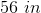, give the length of the smaller base.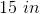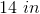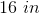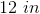Explanation:

Like any polygon, the perimeter of a trapezoid is the total distance around the outside, i.e.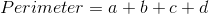,

where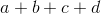are the lengths of each side.

Let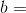small base length and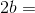big base length.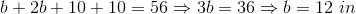### Example Question #2 : Trapezoids

The perimeter of the following trapezoid is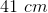.

The following is given: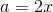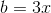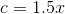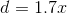Find.

Figure not drawn to scale.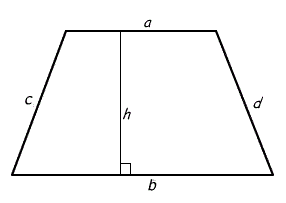Explanation:

The perimeter of a trapezoid is equal to the sum of all of the sides, i.e.,

where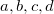are the lengths of each side.